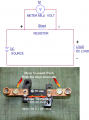# Shunt resistor in DC Amp Meter

#### meowsoft

Joined Feb 27, 2021
494
I don't use DC amp meter with shunt resistor before, can I use DC amp meter with shunt by default and bypass shunt resistor ?, also how to choose shunt resistor size ?, and can I there any sample for amp meter with shunt resistor wiring diagram ?
DC amp meter is only have 2 terminals with "hi" and "low" label

#### Ian0

Joined Aug 7, 2020
3,462
Generally, shunts are sold with a current rating and a voltage rating. The voltage rating refers to the meter that is being used (generally 60mV or 75mV) and the current rating refers to the current being measured.
To choose your own resistor, divide 60mV or 75mV by the current.
There are four terminals on the shunt. Two large ones for the current being measured and two small ones for the meter.

#### meowsoft

Joined Feb 27, 2021
494

Joined Jan 15, 2015
6,114
DC ammeters can be had with an internal shunt which in most cases is a meter having a maximum current of 5 to 10 amps. Normally for higher currents a shunt external to the meter is used.In the above example a 0 to 50 mv DC Millivolt meter is used with an external 0.001 Ohm shunt so 0 to 50 amps in this case equals 0 to 50 mv out. The meter is scaled to read out in Amps rather than Volts. While a shunt is generally drawn as a resistor most higher current shunts actually use Maganin as the resistive alloy because of its ability not to change resistance at higher temperatures. In the above image both sides are copper but the center section is Maganin. Since in a parallel circuit the current "splits" the shunt actually shunts the bulk of the current leaving only a small amount to pass through the metering device. Since meters have a very high input impedance the meter in parallel with the shunt will have no significant results on the shunt resistance. In the above circuit the actual meter would be a 50 mv full scale meter and the scale would read 0 to 50 amps.

Ron

•Tonyr1084

#### Juhahoo

Joined Jun 3, 2019
215
I don't use DC amp meter with shunt resistor before, can I use DC amp meter with shunt by default and bypass shunt resistor ?, also how to choose shunt resistor size ?, and can I there any sample for amp meter with shunt resistor wiring diagram ?
DC amp meter is only have 2 terminals with "hi" and "low" label
You need to know what is your wanted max voltage measured from shunt resistor and MAX current for resistor power.

If you have 10mohm resistor and 10A max current, your Voltage reading from the shunt resistor is max 10A * 0.010ohm = 0.1V
Resistor power dissipation P = 0.1V * 10A = 1W

Now you can decide what do you need.